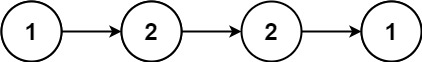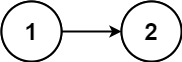## Algorithm

Problem Name: 234. Palindrome Linked List

Given the `head` of a singly linked list, return `true` if it is a palindrome (A palindrome is a sequence that reads the same forward and backward.)or `false` otherwise.

Example 1:```Input: head = [1,2,2,1]
Output: true
```

Example 2:```Input: head = [1,2]
Output: false
```

Constraints:

• The number of nodes in the list is in the range `[1, 105]`.
• `0 <= Node.val <= 9`

## Code Examples

### #1 Code Example with C Programming

```Code - C Programming```

``````
struct ListNode *fast, *slow;
struct ListNode *half = NULL, *tail = NULL;
struct ListNode *p;

while (fast && fast->next && fast->next->next) {
fast = fast->next->next;
slow = slow->next;
}

if (fast && fast->next) {
half = slow->next;
} else {
half = slow;
}

while (half) {
p = half->next;
half->next = tail;
tail = half;
half = p;
}

while (tail) {
if (tail->val != head->val) return false;
tail = tail->next;
}

return true;
}
``````
Copy The Code &

Input

cmd

Output

cmd
true

### #2 Code Example with C++ Programming

```Code - C++ Programming```

``````
class Solution {
public:
while(fast && fast->next){
slow = slow->next;
fast = fast->next->next;
}
if(fast) slow = slow->next; // if odd, move one step forward

ListNode* pre(NULL), *cur(slow), *next;
while(cur){
next = cur->next;
cur->next = pre;
pre = cur;
cur = next;
}

while(pre){
pre = pre->next;
}
return true;
}
};
``````
Copy The Code &

Input

cmd

Output

cmd
true

### #3 Code Example with Java Programming

```Code - Java Programming```

``````
class Solution {
return true;
}
ListNode prev = null;
while (fast != null && fast.next != null) {
prev = slow;
fast = fast.next.next;
slow = slow.next;
}
ListNode revNode = reverse(slow);
while (revNode != null) {
return false;
}
revNode = revNode.next;
}
return true;
}

private ListNode reverse(ListNode node) {
ListNode prev = null;
ListNode curr = node;
ListNode next = node;
while (curr != null) {
next = curr.next;
curr.next = prev;
prev = curr;
curr = next;
}
return prev;
}
}
``````
Copy The Code &

Input

cmd

Output

cmd
false

### #4 Code Example with Javascript Programming

```Code - Javascript Programming```

``````
const arr = []
}
let start = 0
let end = arr.length - 1
while(start < end) {
if(arr[start] !== arr[end]) {
return false
}
start++
end--
}
return true
};
``````
Copy The Code &

Input

cmd

Output

cmd
false

### #5 Code Example with Python Programming

```Code - Python Programming```

``````
class Solution:
l = None
while fast and fast.next:
fast = fast.next.next
r.next, l, r = l, r, r.next
if fast: r = r.next
while l and r and l.val == r.val:
l, r = l.next, r.next
return not l
``````
Copy The Code &

Input

cmd

Output

cmd
true

### #6 Code Example with C# Programming

```Code - C# Programming```

``````
namespace LeetCode
{
{
{
if (head == null) return true;

var secondHalf = ReverseList(endOfFirstHalf.next);

ListNode p1 = head, p2 = secondHalf;
var result = true;
while (p2 != null)
{
if (p1.val != p2.val)
{
result = false;
break;
}

p1 = p1.next;
p2 = p2.next;
}

return result;
}

{
while (fast.next != null && fast.next.next != null)
{
slow = slow.next;
fast = fast.next.next;
}

return slow;
}

{
ListNode prev = null, curr = head;
while (curr != null)
{
var next = curr.next;
curr.next = prev;
prev = curr;
curr = next;
}

return prev;
}
}
}
``````
Copy The Code &

Input

cmd